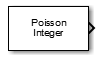# Poisson Integer Generator

Generate Poisson-distributed random integers

## Library

Random Data Sources sublibrary of Comm Sources

•## Description

The Poisson Integer Generator block generates random integers using a Poisson distribution. The probability of generating a nonnegative integer k is

`${\lambda }^{k}\mathrm{exp}\left(-\lambda \right)/\left(k!\right)$`

where λ is a positive number known as the Poisson parameter.

You can use the Poisson Integer Generator to generate noise in a binary transmission channel. In this case, the Poisson parameter Lambda should be less than 1, usually much less.

### Attributes of Output Signal

The output signal can be a column or row vector, a two-dimensional matrix, or a scalar. The number of rows in the output signal corresponds to the number of samples in one frame and is determined by the Samples per frame parameter. The number of columns in the output signal corresponds to the number of channels and is determined by the number of elements in the Lambda parameter. See Sources and Sinks in Communications Toolbox™ User's Guide for more details.

## Parameters

Lambda

The Poisson parameter λ. Specify λ as a scalar or row vector whose elements are real numbers. If Lambda is a scalar, then every element in the output vector shares the same Poisson parameter. If Lambda is a row vector, then the number of elements correspond to the number of independent channels output from the block.

Source of initial seed

The source of the initial seed for the random number generator. Specify the source as either `Auto` or `Parameter`. When set to `Auto`, the block uses the global random number stream.

Note

When Source of initial seed is `Auto` in `Code generation` mode, the random number generator uses an initial seed of zero. Therefore, the block generates the same random numbers each time it is started. Use `Interpreted execution` to ensure that the model uses different initial seeds. If ```Interpreted execution``` is run in `Rapid accelerator` mode, then it behaves the same as ```Code generation``` mode.

Initial seed

The initial seed value for the random number generator. Specify the seed as a nonnegative integer scalar. Initial seed is available when the Source of initial seed parameter is set to `Parameter`.

Sample time

Positive scalars specify the time in seconds between each sample of the output signal. If you set the sample time to `-1`, the output signal inherits the sample time from downstream. For information on the relationship between the Sample time and Samples per frame parameters, see Sample Timing.

Samples per frame

Samples per frame, specified as a positive integer indicating the number of samples per frame in one channel of the output data. For information on the relationship between Sample time and Samples per frame, see Sample Timing.

Output data type

The output type of the block can be specified as a `boolean`, `uint8`, `uint16`, `uint32`, `single`, or `double`. The default is `double`.

Simulate using

Select the simulation mode.

```Code generation```

On the first model run, simulate and generate code. If the structure of the block does not change, subsequent model runs do not regenerate the code.

If the simulation mode is ```Code generation```, System objects corresponding to the blocks accept a maximum of nine inputs.

```Interpreted execution```

Simulate model without generating code. This option results in faster start times but can slow subsequent simulation performance.

expand all

## Version History

Introduced before R2006a

expand all